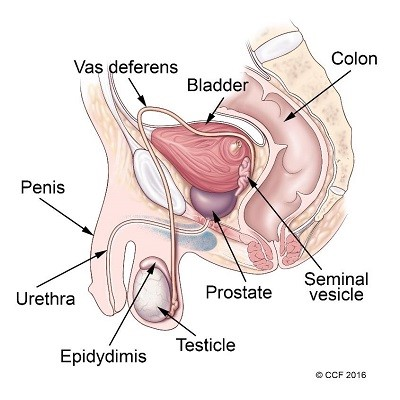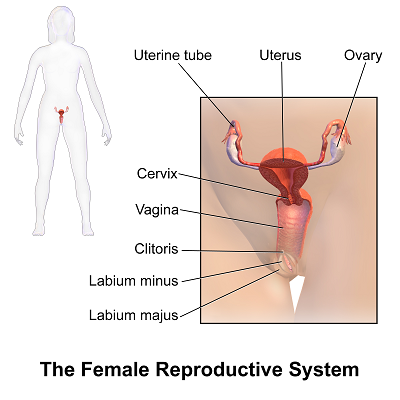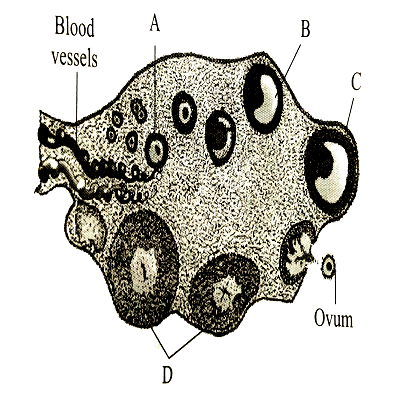# NEET 2023 is Scheduled for 7 May, Check Important Formulas

NEET 2023 will be conducted on 7th May 2023 by NTA. NEET 2023 will be held in offline mode and the exam will be pen and paper-based for all the NEET aspirants. The duration of the exam will be 3 hours & 20 minutes and the total number of questions will be 200 like the previous year’s question paper pattern. NEET 2023 Admit Card is expected to be be released in first week of May 2023.

NEET 2023 question paper has four subjects Chemistry, Physics, Botany, and Zoology. To crack NEET 2023 candidates need to focus on planning and thorough knowledge of all subjects. NEET Important formulas for all subjects are all time beneficial for the candidates. Some of the subject-wise important formulas are listed in the article below. Ca ndidates can make use of this article as last-minute preparation or short notes.

## NEET 2023 Important Formulas of Physics

In NEET 2023 preparation for the exam, a chart of Important Formulas of Physics will be very helpful to all NEET aspirants. Every time it’s very difficult to remember all the Physics formulas from different chapters. So topic-wise important formulas of Physics for NEET 2023 are listed below:

Important Formulas

• Gravitation constant G = 6.67×10−11 N m2/kg2 ​​
• Planck constant h = 6.63 × 10−34s = 4.136 × 10-15 eV.s
• Boltzmann constant k = 1.38 × 10−23 J/K
• Avogadro’s number NA = 6.023 × 1023 mol−1
• Molar gas constant R = 8.314 J/(mol K)
• Charge of electron e = 1.602 × 10−19 C
• Coulomb constant 1/4πε0 = 8.9875517923(14) × 109 N m2/C2
• Permittivity of vacuum 0 = 8.85 × 10−12 F/m
• Faraday constant F = 96485 C/mol
• Mass of electron me = 9.1 × 10−31 kg
• Mass of proton mp = 1.6726 × 10−27 kg
• Mass of neutron mn = 1.6749 × 10−27 kg
• Rydberg constant R∞ = 1.097 × 107 m−1
• Bohr magneton µB = 9.27 × 10−24 J/T
• Bohr radius a0 = 0.529 × 10−10 m
• Standard atmosphere atm = 1.01325 × 105 Pa
• Wien displacement constant b = 2.9 × 10−3 m K.
• Wave = ∆x ∆t wave = average velocity ∆x = displacement ∆t = elapsed time.
• Stefan-Boltzmann constant σ = 5.67 × 10−8 W/(m2 K4)
• Vavg = (vi + vf*)2

Important Symbols

• a = ∆v ∆t,

A = acceleration

∆v = change in velocity

∆t = elapsed time.

• ∆x = vi∆t + 1/2 a(∆t)2

∆x = the displacement

vi = the initial velocity

∆t = the elapsed time

a = the acceleration

Use this formula when you don’t have vf.

• ∆x = vf∆t − 1/2 a(∆t)2

∆x = displacement

vf = is the final velocity

∆t = elapsed time

a = acceleration

Use this formula when you don’t have vi.

• F = ma

F = force

m = mass

Then a = acceleration Newton’s Second Law.

F is the net force on the mass m.

• W = mg

W = weight

m = mass

g = acceleration which is due to gravity.

Then we see that the weight of an object with mass m. This is said to be really just Newton’s Second Law.

• f = µN f = friction force

µ = coefficient of friction

N = normal force

Here µ can be either the kinetic coefficient of friction µk or the static coefficient of friction.

• p = mv
• W = F d cos θ

W = work t

F = force

d = distance

θ = angle between F and the direction of motion

• KE = 1/2 mv2 K

KE = kinetic energy

m = mass

v = velocity

• PE = mgh

PE = potential energy

m = mass

g = acceleration due to gravity

h = height

• W = ∆(KE)

W = work done

KE = kinetic energy.

The “work energy” that we have learned is the theorem that the work done by the net force on an object equals the change in kinetic energy of the object.

We can write it as E = KE + PE

E = total energy

KE = kinetic energy

PE = potential energy

## NEET 2023 Important Formulas of Chemistry

Chemistry is one of the main subjects in NEET 2023. Listed below are some  Important Formulas of Chemistry of NEET 2023 which will be very helpful in the preparation of the exam.

• Ideal Gas Law: PV=nRT
• Boyle’s Law: PV=k

(constant at constant temperature)

• Charles’s Law: VT=k

(constant at constant pressure)

• Gay-Lussac’s Law: PT=k

(constant at constant volume)

• Henry’s Law: C=kP

(concentration of gas in solution)

• Rate of Reaction: Rate =k[A]m[B]n
• pH Scale: pH=−log[H+]
• Acid Dissociation Constant: Ka=[H+][A−][HA]
• Base Dissociation Constant: Kb=[OH−][B+][BOH]
• Henderson-Hasselbalch Equation: pH=pKa+log([A−][HA])
• Nernst Equation: E=E∘−(0.0592n)log(Q)
• Gibbs Free Energy: ΔG=ΔH−TΔS
• Arrhenius Equation: k=Ae(−EaRT)
• Mole Fraction: Mole Fraction =Number of moles of componentTotal number of moles of all components
• Molarity: Molarity (M) =Number of moles of soluteVolume of solution in liters

## NEET 2023 Important Formulas of Biology

In NEET 2023 Biology paper has two sections Botany and Zoology. NEET 2023 carries a total of 720 marks and only the Biology paper carries 360 marks weightage in NEET. A total of 90 questions were there of which 45 is for Botany and 45 is for Zoology. Candidates must prepare a chart of diagrams and important names and important chapters.

Important Chapters of NEET 2023

• Biological Classification
• Morphology of flowering plants
• Molecular Basis of Inheritance
• Principles of Inheritance and Variation
• Animal Kingdom
• Sexual Reproduction in Flowering Plants
• Human Reproduction

Diagram of Male Reproductive SystemDiagram Female Reproductive SystemDiagram of Sectional View of OvaryThese are some useful terms & formulas for NEET 2023 Preparation. Based on the previous year-wise NEET paper analysis, we have collected this information. Hope this is useful for you. All the Best!### Technonguide

Technonguide is an IOT guide for Latest technology News, Trends, and Updates for professionals in digital marketing, social media, web analytics, content marketing, digital strategy.